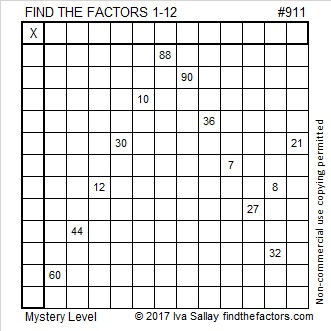# 911 Mystery Level Puzzle

911 is a prime number that is also the sum of three consecutive primes:

• 293 + 307 + 311 = 911

911 is 191 in BASE 26 because 1(26²) + 9(26) + 1(1) = 911

911 is palindrome 12121 in BASE 5 because 1(5⁴) + 2(5³) + 1(5²) + 2(5) + 1(1) = 911Print the puzzles or type the solution on this excel file: 12 factors 905-913

• 911 is a prime number.
• Prime factorization: 911 is prime.
• The exponent of prime number 911 is 1. Adding 1 to that exponent we get (1 + 1) = 2. Therefore 911 has exactly 2 factors.
• Factors of 911: 1, 911
• Factor pairs: 911 = 1 × 911
• 911 has no square factors that allow its square root to be simplified. √911 ≈ 30.1827765

How do we know that 911 is a prime number? If 911 were not a prime number, then it would be divisible by at least one prime number less than or equal to √911 ≈ 30.2. Since 911 cannot be divided evenly by 2, 3, 5, 7, 11, 13, 17, 19, 23, or 29, we know that 911 is a prime number.This site uses Akismet to reduce spam. Learn how your comment data is processed.